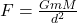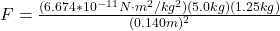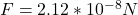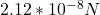## Kramer goes bowling and decides to employ the force of gravity to “pick up a spare.” He rolls the 5.00 kg bowling ball very slowly so that i

Question

Kramer goes bowling and decides to employ the force of gravity to “pick up a spare.” He rolls the 5.00 kg bowling ball very slowly so that it comes to rest a center-to-center distance of 0.140 m from the one remaining 1.25 kg bowling pin. Treat both the ball and the pin as point objects, and determine the magnitude of the force of gravity Fgrav between them.

in progress 0
7 months 2021-07-22T15:39:37+00:00 1 Answers 9 views 0

1. To solve this problem we will apply the concepts related to the gravitational force expressed in Newton’s statements as the product between the constant of gravity, the two masses to study and the distance to the square that separates them. Mathematically this can be described asHere,

G = Gravitational constant

m = Mass 1

M =Mass 2

d = Distance between them

Replacing with our values,Therefore the force of gravity is